Courses

# Couette Flow - Viscous Incompressible Flows Mechanical Engineering Notes | EduRev

## Mechanical Engineering : Couette Flow - Viscous Incompressible Flows Mechanical Engineering Notes | EduRev

The document Couette Flow - Viscous Incompressible Flows Mechanical Engineering Notes | EduRev is a part of Mechanical Engineering category.
All you need of Mechanical Engineering at this link: Mechanical Engineering

Couette Flow

Couette flow is the flow between two parallel plates (Fig. 26.1). Here, one plate is at rest and the other is moving with a velocity . Let us assume the plates are infinitely large in direction, so the dependence is not there.

The governing equation is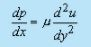flow is independent of any variation in z-direction.
The boundary conditions are ---(i)At y = 0, u = 0 (ii)At y = h, u = U.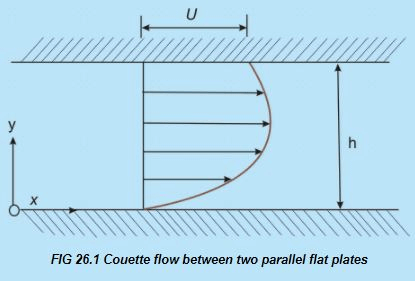• We get,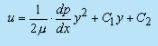Invoking the condition (at y = 0, u = 0) C2   becomes equal to zero.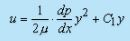Invoking the other condition (at y = h, u = U)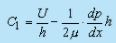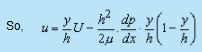(26.1)

Equation (26.1) can also be expressed in the form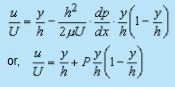(26.2a)

Where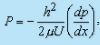Equation (26.2a) describes the velocity distribution in non-dimensional form across the channel with P as a parameter known as the non-dimensional pressure gradient .

• When P = 0, the velocity distribution across the channel is reduced to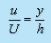This particular case is known as simple Couette flow.

• When P > 0 , i.e. for a negative or favourable pressure gradient (-dp/ dx) in the direction of motion, the velocity is positive over the whole gap between the channel walls. For negative value of P ( P < 0 ), there is a positive or adverse pressure gradient in the direction of motion and the velocity over a portion of channel width can become negative and back flow may occur near the wall which is at rest. Figure 26.2ashows the effect of dragging action of the upper plate exerted on the fluid particles in the channel for different values of pressure gradient.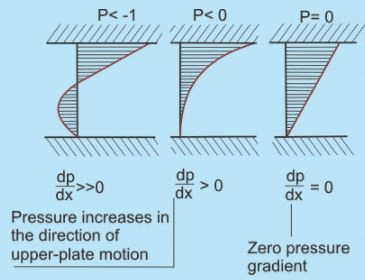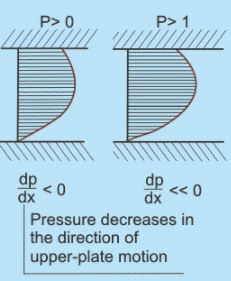FIG 26.2a - Velocity profile for the Couette flow for various values of pressure gradient

Maximum and minimum velocities

The quantitative description of non-dimensional velocity distribution across the channel, depicted by Eq. (26.2a), is shown

in Fig. 26.2b.

• The location of maximum or minimum velocity in the channel is found out by setting du/ dy =0. From Eq. (26.2a), we can write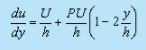Setting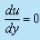gives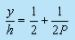(26.2b)

• It is interesting to note that maximum velocity for P = 1 occurs at y/h = 1 and equals to . For P > 1, the maximum velocity occurs at a location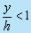.
• This means that with P > 1, the fluid particles attain a velocity higher than that of the moving plate at a location somewhere below the moving plate.
• For P = -1, the minimum velocity occurs,  at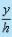= 0. For P < -1,  the minimum velocity occurs at a location> 1.
• This means that there occurs a back flow near the fixed plate. The values of maximum and minimum velocities can be determined by substituting the value of from Eq. (26.2b) into Eq. (26.2a) as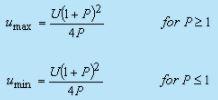(26.2b)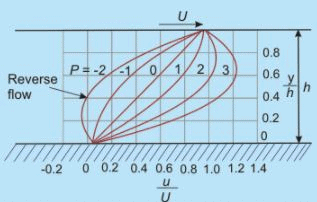FIG 26.2b - Velocity distribution of the Couette flow

Hagen Poiseuille Flow

• Consider fully developed laminar flow through a straight tube of circular cross-section as in Fig. 26.3. Rotational symmetry is considered to make the flow two-dimensional axisymmetric.
• Let us take z-axis as the axis of the tube along which all the fluid particles travel, i.e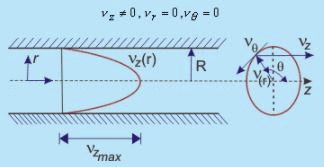Fig 26.3 - Hagen-Poiseuille flow through a pipe

• Now, from continuity equation, we obtain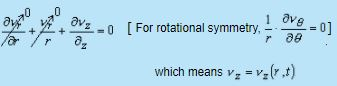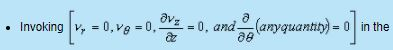Navier-Stokes equations, we obtain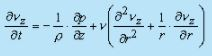(in the z-direction)             (26.3)

• For steady flow, the governing equation becomes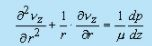(26.4)

The boundary conditions are- (i) At r = 0, vis finite and (ii) r = R, v= 0 yields

• Equation (26.4) can be written as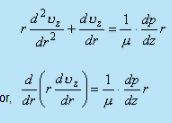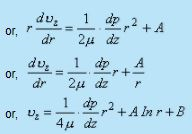• At r =0,  v is finite which means A should be equal to zero and at r = R,  v= 0 yields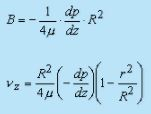(26.5)

• This shows that the axial velocity profile in a fully developed laminar pipe flow is having parabolic variation along r.

• At r = 0, as such, v= vmax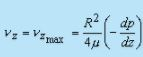(26.6a)

• The average velocity in the channel,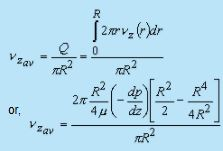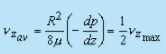(26.6b)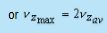(26.6c)

• Now, the discharge (Q) through a pipe is given by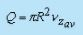(26.7)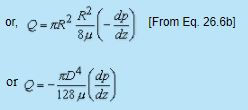(26.8)

Applications-

• Equation (26.8) is commonly used in the measurement of viscosity with the help of capillary tube viscometers . Such a viscometer consists of a constant head tank to supply liquid to a capillary tube (Fig. 26.4).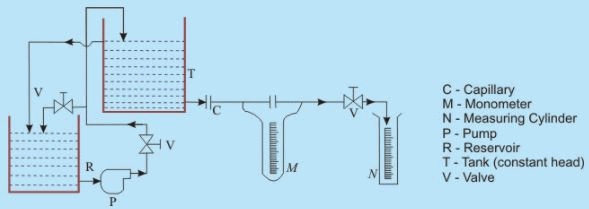FIG 26.4 Schematic diagram of the experimental facility for determination of viscosity

• Pressure drop readings across a specified length in the developed region of the flow are taken with the help of a manometer. The developed flow region is ensured by providing the necessary and sufficient entry length.
• From Eq. (26.8), the expression for viscosity can be written as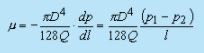• The volumetric flow rates (Q) are measured by collecting the liquid in a measuring cylinder. The diameter (D) of the capillary tube is known beforehand. Now the viscosity of a flowing fluid can easily be evaluated.
• Shear stress profile across the cross-section can also be determined from this information. Shear stress at any point of the pipe flow is given by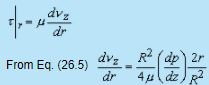(26.9a)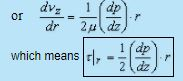(26.9b)

This also indicates that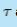varies linearly with the radial distance from the axis.

• At the wall,assumes the maximum value.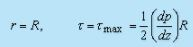Again, over a pipe length of , the total shear force is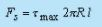or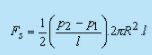or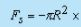[Pressure drop between the specified length]

as it should be. Negative sign indicates that the force is acting in opposite to the flow direction.

• However, from Eq. (26.6b), we can write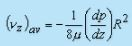(26.9c)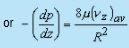(26.10)

Losses and Friction Factors

Over a finite length l , the head loss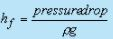(26.11)

Combining Eqs (26.10) and (26.11), we get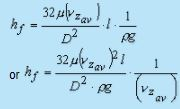(26.12)

• On the other hand, the head loss in a pipe flow is given by Darcy-Weisbach formula as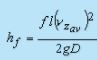(26.13)

where "f" is Darcy friction factor . Equations (26.12) and (26.13) yieldwhich finally gives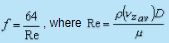is the Reynolds number.

• So, for a fully developed laminar flow, the Darcy (or Moody) friction factor is given by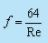(26.14a)

Alternatively, the skin friction coefficient for Hagen-Poiseuille flow can be expressed by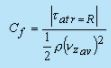With the help of Eqs (26.9b) and (26.9c), it can be written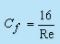(26.14b)

The skin friction coefficient Cf is called as Fanning's friction factor . From comparison of Eqs (26.14a) and (26.14b), it appears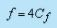• For fully developed turbulent flow, the analysis is much more complicated, and we generally depend on experimental results. Friction factor for a wide range of Reynolds number (104 to 108) can be obtained from a look-up chart . Friction factor, for high Reynolds number flows, is also a function of tube surface condition. However, in circular tube, flow is laminar for Re ≤ 2300 and turbulent regime starts with Re ≥ 4000.
• The surface condition of the tube is another responsible parameter in determination of friction factor.
• Friction factor in the turbulent regime is determined for different degree of surface-roughness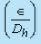of the pipe, where ∈ is the dimensional roughness and Dh is usually the hydraulic diameter of the pipe .
• Friction factors for different Reynolds number and surface-roughness have been determined experimentally by various investigators and the comprehensive results are expressed through a graphical presentation which is known as Moody Chart after L.F. Moody who compiled it.
• The hydraulic diameter which is used as the characteristic length in determination of friction factor, instead of ordinary geometrical diameter, is defined as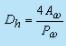(26.15)

where Aw is the flow area and Pw is the wetted perimeter

Kinetic energy correction factor α The kinetic energy associated with the fluid flowing with its profile through elemental area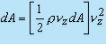and the total kinetic energy passing through per unit time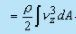This can be related to the kinetic energy due to average velocity(v zav), through a correction factor, α as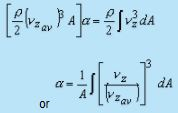Here, for Hagen-Poiseuille flow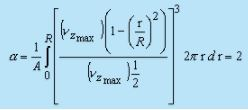(26.16)

Flow between Two Concentric Rotating Cylinders

•  Another example which leads to an exact solution of Navier-Stokes equation is the flow between two concentric rotating cylinders.
• Consider flow in the annulus of two cylinders (Fig. 26.5), where r1 and r2 are the radii of inner and outer cylinders, respectively, and the cylinders move with different rotational speeds ω1 and ω2 respectively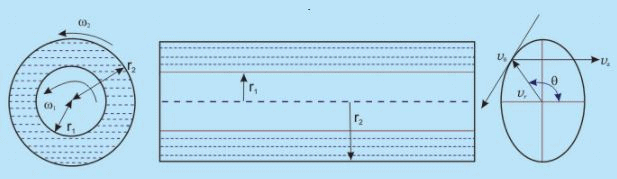FIG 26.5 - Flow between two concentric rotating cylinders

• From the physics of the problem we know, .vz = 0, vr = 0
• From the continuity Eq. and these two conditions, we obtain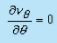which means vθ is not a function of θ. Assume dimension to be large enough so that end effects can be neglected and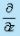(any property) = 0.

• This implies  vθ  = vθ (r). With these simplifications and assuming that " θ symmetry" holds good, Navier-Stokes equation reduces to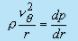(26.17)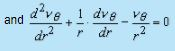(26.18)

• Equation (26.17) signifies that the centrifugal force is supplied by the radial pressure, exerted by the wall of the enclosure on the fluid. In other words, it describes the radial pressure distribution.
From Eq. (26.18), we get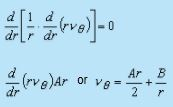(26.19)

• For the azimuthal component of velocity, vθ, the boundary conditions are: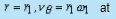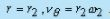• Application of these boundary conditions on Eq. (26.19) will produce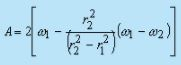and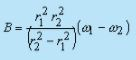• Finally, the velocity distribution is given by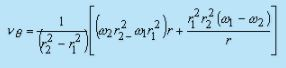(26.20)

Calculation of Stress and Torque Transmitted

Now,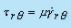is the general stress-strain relation.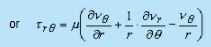• In our case,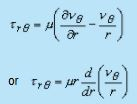(26.21)

• Equations (26.20) and (26.21) yields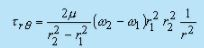(26.22)

• Now,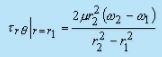and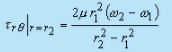• For the case, when the inner cylinder is at rest and the outer cylinder rotates, the torque transmitted by the outer cylinder to the fluid is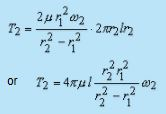(26.23)

where is the length of the cylinder.

• The moment T1, with which the fluid acts on the inner cylinder has the same magnitude. If the angular velocity of the external cylinder and the moment acting on the inner cylinder are measured, the coefficient of viscosity can be evaluated by making use of the Eq. (26.23)
Offer running on EduRev: Apply code STAYHOME200 to get INR 200 off on our premium plan EduRev Infinity!

,

,

,

,

,

,

,

,

,

,

,

,

,

,

,

,

,

,

,

,

,

;